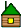Valery Zamiralov

## Introduction to group theory and its representations and some applications to particle physics

• Introduction
• Groups and algebras. Basic notions
• Representations of the Lie groups and algebras
• Unitary unimodular group SU(2)
• SU(2) as a spinor group
• Isospin group SU(2)
• Unitary symmetry group SU(3)
• Eightfold way. Mass formulae in SU(3)
• Baryon and meson unitary multiplets
• Mass formulae for octet of pseudoscalar mesons
• Mass formulae for octet of  baryons JP = 1/2+
• Nonet of the vector meson and mass formulae
• Decuplet of baryon resonances with  JP = 3/2+ and its mass formulae
• Praparticles and hypothesis of quarks
• Quark model. Mesons in quark model
• Charm and its arrival in particle physics
• The fifth quark. Beauty
• Truth or top?
• Baryons in quark model
• Electromagnetic current in the models of unitary symmetry and of quarks
• On magnetic moments of barions
• Radiative decays of  vector mesons
• Leptonic decays of vector mesons V--> l+l-
• Photon as a gauge field
• Rho-meson as a gauge field
• Vector and axial-vector currents in unitary symmetry and quark model
• General remarks on weak interaction
• Weak currents in unitary symmetry model
• Weak currents in a quark model
• Neutral weak currents
• GIM model
• Construction of the Salam-Weinberg model
• Six quark model and CKM matrix
• Vector bosons W and Y as gauge fields
• About Higgs mechanism
• Colour and its appearence in particle physics
• Gluon as a gauge field
• Simple examples with coloured quarks

VI. Conclusion